# Related Videos

## Solve the quadratic equation $32x^2-18x-17=0$

Go!
1
2
3
4
5
6
7
8
9
0
a
b
c
d
f
g
m
n
u
v
w
x
y
z
.
(◻)
+
-
×
◻/◻
/
÷
2

e
π
ln
log
log
lim
d/dx
Dx
|◻|
=
>
<
>=
<=
sin
cos
tan
cot
sec
csc

asin
acos
atan
acot
asec
acsc

sinh
cosh
tanh
coth
sech
csch

asinh
acosh
atanh
acoth
asech
acsch

### Videos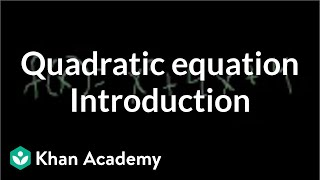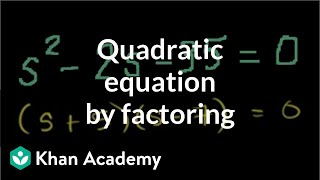### Solving a quadratic equation by factoring | Algebra II | Khan Academy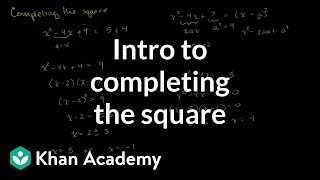### Solving quadratic equations by completing the square | Algebra II | Khan Academy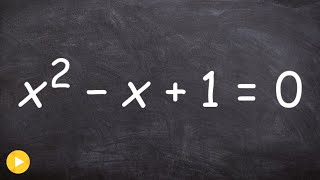### Solve using the quadratic formula with complex solutions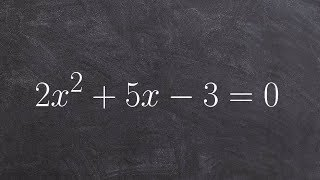### How to solve a quadratic equation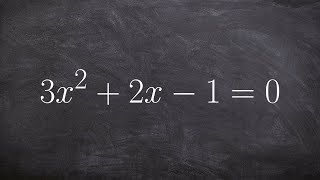### Algebra 2 - Solving by completing the square and factoring out a three 3x^2+2x-1=0

$32x^2-18\cdot x-17=0$